# Mathematics

## Logarithms

### Exponential Functions

A function in the form of y = ax, where a >= 1 is called an exponential function.

E.g.

y = 2x; y = 3x

The following image shows the nature of two exponential functions: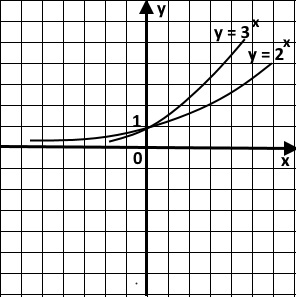Exponential functions show the following features, regardless of the number:

• They go through (0,1) on a grid.
• For a small increase in x, there is a steep increase in y - hence, the change becomes exponential.
• As x approaches negative infinity, y approaches 0 - creating an asymptote.
• The values of the functions remain positive, regardless of the value of x.

### The Exponential Function - ex

This is a special exponential function, which is widely used in mathematics. It's special, because the gradient of the curve at any point is the same as the value of the function at the same point. It's still an exponential function, because it shows all the features that we discussed before.

The following image shows this feature: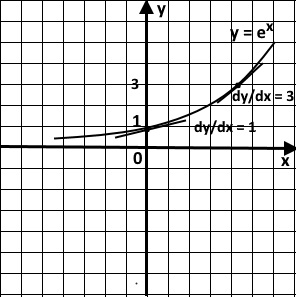### The Exponential Function - ex - and the Natural Logarithm Function - ln(x)

The following image shows the exponential function and the natural logarithm function on the same grid. You can see they are closely related and worth studying together.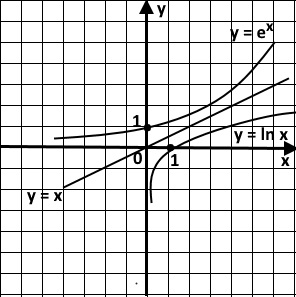The following features can be seen in the two graphs:

• They swap around x and y values - e0 = 1; ln(1) = 0.
• They are symmetrical around y = x line.
• ln(x) is not defined for x = 0 or negative x values.
• The values of the function ln(x) can be positive, negative or even 0.

### Logarithms

Consider the following indices and how they produce the corresponding logarithm values:

82 = 64 => we say that log864 = 2; - this is read as log 64 to the base 8 is 2.

43 = 64 => we say that log464 = 3; - this is read as log 64 to the base 4 is 3.
Note the positions of the bases and indices in each case.

You may have noticed that in both cases the function is log(64), yet the values are different, because of the different bases. It shows the significance of the base, when it comes to dealing with logarithms. In short, log without a base is like a laptop without a keypad! The base is really important in logarithms.

In theory, a logarithm function can take any base value; the most used bases, however, are 10 and e.
log10x = log(x) or lg(x); logex = ln(x);

Let's solve some problems involving logarithms now; the following approach is highly recommended to master this topic:

E.g.1

Find the value of log327
Let log327 = x
3x = 27
Now write down 27 in index form with the same base.
3x = 33
So, x = 3.
log327 = 3.

E.g.2

Find the value of log232
Let log232 = x
2x = 32
Now write down 32 in index form with the same base.
2x = 25
So, x = 5.
log232 = 5.

E.g.3

Find the value of log10100
Let log10100 = x
10x = 100
Now write down 100 in index form with the same base.
10x = 102
So, x = 2.
log10100 = 2.

E.g.4

Find the value of log22
Let log22 = x
2x = 2
Now write down 2 in index form with the same base.
2x = 21
So, x = 1.
log22 = 1.

E.g.5

Find the value of log31
Let log31 = x
3x = 1
Now write down 1 in index form with the same base.
3x = 30
So, x = 0.
log31 = 0.

E.g.6

Find the value of log21/2
Let log21/2 = x
2x = 1/2
Now write down 1/2 in index form with the same base.
2x = 2-1
So, x = -1.
log21/2 = -1.

E.g.7

Find the value of log48
Let log48 = x
4x = 8
Now write down both 4 and 8 in a common base, such as 2, in index form.
(22)x = 23
22x = 23
So, 2x = 3.
x = 3/2
log48 = 3/2.

E.g.8

Find the value of log366
Let log366 = x
36x = 6
Now write down both in index form with the base 6.
36x = 61
(62)x = 61
So, 62x = 61
2x = 1 => x = 1/2
log366 = 1/2.

E.g.9

Find the value of log0.254
Let log0.254 = x
0.25x = 4
Now write down both in index form with the base 4.
(1/4)x = 41
(4-1)x = 41
So, 4-x = 41
-x = 1 => x = -1
log0.254 = -1.

E.g.10

Find the value of log168
Let log168 = x
16x = 8
Now write down both in index form with the base 2.
(24)x = 23
So, 24x = 23
4x = 3 => x = 3/4
log168 = 3/4.

E.g.11

Solve logx125 = 3
x3 = 125
Now write down 125 with the base 5.
x3 = 53
x = 5

E.g.12

Solve logx9 = 0.5
x1/2 = 9
Now write down 9 with the base 81.
x1/2 = 811/2
x = 81

Laws of Indices

Indices and logarithms go hand in hand. So, it's really important to understand the rules of indices, in order to deal with logarithms. The following examples may be helpful, when it comes to revising the basic rules of indices:

1. ax . ay = ax + y

E.g.
23 . 24 = 23 + 4 = 27

2. ax / ay = ax - y

E.g.
27 / 24 = 27 - 4 = 23

3. (ax)y = axy

E.g.
(23)2 = 23X2 = 26

### Laws of Logarithms

1. loga(xy) = logax + logay

Proof:
Let loga(x) = k and loga(y) = l
So, ak = x and al = y
By multiplying together, xy = ak X al = ak + l
loga(xy) = k + l = loga(x) + loga(y).

E.g.

Find loga(4X3).
loga(4X3) = loga4 + loga3.

2. loga(x/y) = logax - logay

Proof:
Let loga(x) = k and loga(y) = l
So, ak = x and al = y
By dividing, x/y = ak :- al = ak - l
loga(x/y) = k - l = loga(x) - loga(y).

E.g.

Find loga(5/2).
loga(5/2) = loga5 - loga2.

3. loga(x)y = ylogax

Proof:
Let loga(x) = k
ak = x
(ak)y = xy
aky = xy
loga(xy) = ky
loga(xy) = yloga(x).

E.g.

Find loga(4)3.
loga(4)3 = 3loga4.

Proof:
loga(1/x) = loga(1) - loga(x)
= 0 - loga(x)
- loga(x).

E.g.

Express loga(1/4) in an alternate form.
loga(1/4) = -loga4.

Proof:
Let logb(x) = k
bk = x
Taking log to the base a on both sides,
loga(bk) = loga(x)
k loga(b) = loga(x)
k = loga(x)/loga(b)
logb(x) = loga(x)/loga(b).

E.g.

Find log4(8) as a logarithm of the base 2.
log48 = log28/log24
Let x = log28 => 2x = 8 => x = 3
Let y = log24 => 2y = 4 => y = 2
log48 = 3/2

Proof:
logx(y) = logy(y)/logy(x)
= 1/logy(x).

E.g.

Express log4(8) in an alternate form.
log4(8) = 1/log84.

Logarithms Problem Solving

E.g.1

Write log38 + log37 as a single logarithm.
log38 + log37 = log38X7
log356.

E.g.2

Write log35 + log34 - log32 as a single logarithm.
log35 + log34 - log32 = log3(5X4/2)
log310.

E.g.3

Simplify log381 - log39
log381 - log39 = log3(81/9)
= log39
Let x = log39
3x = 9 = 32
x = 2
log381 - log39 = 2.

E.g.4

Simplify 2 log39 - log327
2 log39 - log327 = log392 - log327
= log3(81/27)
= log33
Let x = log33
3x = 3 = 31
x = 1
2 log39 - log327 = 1.

E.g.5

Simplify 3 log35 - 2 log35
3 log35 - 2 log35 = log353 - log352
= log3(125/25)
= log35.

E.g.6

Simplify 3 log66 - 2 log66
3 log66 - 2 log66 = log663 - log662
= log6(216/36)
= log66
Let x = log66
6x = 6 = 61
x = 1
3 log66 - 2 log66 = 1.

E.g.7

Simplify 3 log44 + 1/2 log44 - 2 log44
3 log44 + 1/2 log44 - 2 log44 = log443 + log441/2 - log442
= log4(64X2/16)
= log48
Let x = log48
4x = 8
(22)x = 23
2x = 3 => x = 3/2
3 log44 + 1/2 log44 - 2 log44 = 3/2.

E.g.8

Simplify 4 log1010 - (2 log105 + 2 log102)
4 log1010 - (2 log105 + 2 log102 = log10104 - (log1052 + log1022)
= log10(10000/25X4)
= log10100
Let x = log10100
10x = 100
10x = 102
x = 2
4 log1010 - (2 log105 + 2 log102) = 2.

E.g.9

Write in terms of logxa, logxb and logxc, the simplified term of logx(a2b / √c).
logx(a2b / √c) = logxa2 + logxb - logx√ c
= 2 logxa + logxb - 1/2 logxc

E.g.10

Write in terms of logxa and logxb, the simplified term of logx(a2b2).
logx(a2b2) = logxa2 + logxb2
= 2 logxa + 2 logxb
= 2(logxa + logxb).

### Solving equations involving logarithms

E.g.1

Solve 10x = 102.
Before finding the value of x, we can estimate it; since 102 = 100, x must be slightly bigger than 2.
10x = 102 => log10102 = x
From calculator, log10102 = 2.009
So, x = 2.009(3 d.p.).

E.g.2

Solve 102x - 1 = 400.
102x - 1 = 400 => log10400 = 2x - 1
From calculator, log10400 = 2.6
So, 2x - 1 = 2.6
2x = 3.6
x = 1.8

E.g.3

Solve 5x = 130.
Since 53 = 125, x must be slightly bigger than 3. Let's solve this by logarithms of different bases.
Method 1 - using log to the base 10
log105x = log10130
x log105 = 10130
x = 10130/105
= 3.02(2dp)
Method 2 - using log to the base e
loge5x = loge130
x loge5 = loge130
x ln(5) = ln(130)
x = ln(130)/ln(5)
= 3.02(2 d.p.)
The answer is almost the same regardless of the log base.

E.g.4

Solve 5(2x + 1) = 3(x - 1) .
log(52x + 1) = 3(x - 1)
(2x + 1)log 5 = (x - 1)log 3
(2x + 1)/(x - 1) = log 3 / log 5
(2x + 1)/(x - 1) = log 3 / log 5 = 0.6826
2x + 1 = 0.6826x - 0.6826
1.3174x = -1.6826
x = -1.28

E.g.5

Solve 32x + 4(3x) - 12 = 0.
Let y = 3x => 32x = 3x . 3x = y2
So, the equation becomes, y2 + 4y - 12 = 0
(y + 6)(y - 2) = 0
y = -6 or y = 2
Since, y, 3x - an exponential function - cannot be negative,
y = 2 => 3x = 2
log 3x = log 2
x log 3 = log 2
x = log 2/ log 3
x = 0.63(2 d.p.)

E.g.6

log3x + 8/log3x = 6
Let y = log3x
y + 8/y = 6
y2 + 8 = 6y
y2 - 6y + 8 = 0
(y - 4)(y - 2)=0
y = 4 or y = 2
log3x = 4 or log3x = 2
x = 34 or x = 32
x = 81 or x = 9

E.g.6

log5(3 - 2x) = log25(5x2 - 13x + 3)
= log5(5x2 - 13x + 3) / log525
Since log525 = 2,
log5(5x2 - 13x + 3) / log525 = log5(5x2 - 13x + 3) / 2
2 log5(3 - 2x) = log5(5x2 - 13x + 3)
log5(3 - 2x)2 = log5(5x2 - 13x + 3)
log5(3 - 2x)2 - log5(5x2 - 13x + 3) = 0
log5[(3 - 2x)2 / (5x2 - 13x + 3)] = 0
Since log(1) = 0 => [(3 - 2x)2 / (5x2 - 13x + 3)] = 1
9 - 12x + 4x2 = 5x2 - 13x + 3
x2 - x - 6 = 0
(x - 3)(x + 2) = 0
x = 3 or x = -2
Since x = 3 is not valid for log(3 - 2x),
x = -2

### Sketching Straight Lines from Curves

Thanks to logarithms, a complex mathematical relationship can be sketched as straight line, after a transformation in the format. In practice, it is tremendously useful and easy to analyse the relationship.

Suppose, the relationship involved is y = xn, where n is a constant.
So, log y = log xn
log y = n log x
This is in the form of y = mx, which is a straight line, as follows: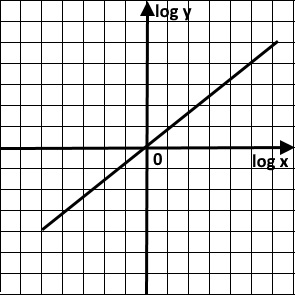In the same way, we can turn the following curves into straight lines easily by converting the variables into logarithms.
y = x2 => log y = 2 log x
y = x3 => log y = 3 log x
y = x4 => log y = 4 log x
The gradient of each line is the index number of each function of the corresponding curve. The straight lines are as follows: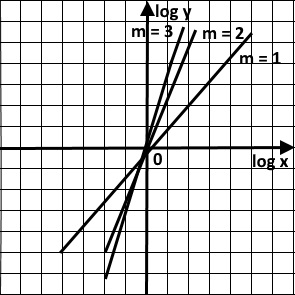In addition, even a complex relationship can be turned into a corresponding logarithm function.

Suppose, y = pxn
log y = log pxn
log y = log p + log xn
log y = log p + n log x
log y = n log x + log p
y = mx + c
The line is as follows: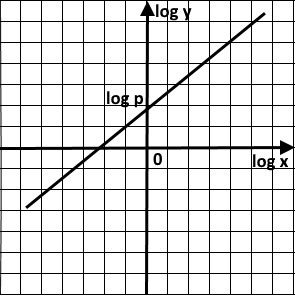The gradient, m = n; y-intercept = log p

Do you want this tutorial with more worked examples? It's available at Amazon:Maths is challenging; so is finding the right book. K A Stroud, in this book, cleverly managed to make all the major topics crystal clear with plenty of examples; popularity of the book speak for itself - 7th edition in print.

### Recommended - GCSE & iGCSEThis is the best book available for the new GCSE(9-1) specification and iGCSE: there are plenty of worked examples; a really good collection of problems for practising; every single topic is adequately covered; the topics are organized in a logical order.

### Recommended for A LevelThis is the best book that can be recommended for the new A Level - Edexcel board: it covers every single topic in detail;lots of worked examples; ample problems for practising; beautifully and clearly presented.